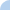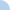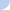# 2014 Algebra Semester 1 Final

Show mastery of the following skills: 1) Graph a linear function (on paper) 2) Order of Operations 3) Evaluate expressions with variables 4) Calculate with fractions 5) Multi-step equations 6) Calculate slope 7) Write a linear equation 8) Solve a system with substitutionFirst name Last nameThis activity was created by a Quia Web subscriber. Learn more about QuiaCreate your own activities